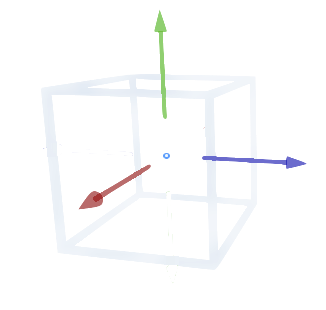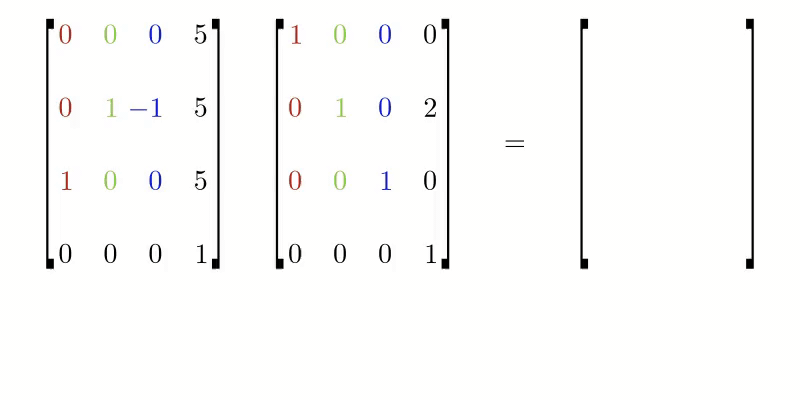## Roblox CFrameIn Roblox, a CFrame (coordinate frame) is an object that encodes position and rotation in 3D space.

You can create a CFrame with the new() constructor, which accepts a set of 3d coordinates:

local cframe = CFrame.new(0, 10, 0)


CFrame is a property of Roblox Part. You can move a Part by assigning a new the CFrame to it:

local part = Instance.new('Part')
part.CFrame = CFrame.new(0, 10, 0)


Note that it's the same as assigning a Roblox Vector3 to the Position property.

part.Position = Vector3.new(0, 10, 0)


However, CFrame can also include information about a Part's rotation.

You can also construct a rotated CFrame using the Angles constructor. It takes the rotation around each axis in Radians:

part.CFrame = CFrame.Angles(0, math.rad(90), 0)


That's the same as assigning a rotational Vector3 to the Orientation property (although here we pass angles in degrees):

part.Orientation = Vector3.new(0, 90, 0)


You can combine a position CFrame with an Angles CFrame by multiplying them together:

--- Place a part at 0, 10, 0 with Orientation 0, 90, 0.
part.CFrame = CFrame.new(0, 10, 0) * CFrame.Angles(0, math.rad(90), 0)


Later in the article, we'll look at what is happening under the hood when multiplying two CFrames.

CFrame has many handy constructor methods.

You can create a CFrame that looks at another Part using the lookAt constructor:

local thing = game.Workspace.Thing
local position = Vector3.new(0, 0, 0)

part.CFrame = CFrame.lookAt(position, thing)


A CFrame can be used to calculate relative position from CFrame. For example, if I want to put a Part in front of a player's face, no matter which direction they're facing (even if they're upside down), we can use the ToWorldSpace() method:

local relativePositionOfNewPart = CFrame.new(0, 0, 10)


That method is the equivalent of multiplying the left CFrame by the right character.Head.CFrame * relativePositionOfNewPart (note that CFrame multiplication is not communitive - the order matters).

A CFrame is composed of 4 Vectors.

1. Position vector $(\mathbf{x}, \mathbf{y}, \mathbf{z})$
2. Right vector $(rX, rY, rZ)$
3. Up vector $(uX, uY, uZ)$
4. Look (front) vector $(lX, lY, lZ)$

The right, up, and look vectors are perpendicular vectors that describe the CFrame's rotation.

You can also access each vector using their respective properties:

print(CFrame.Position)
print(CFrame.RightVector)
print(CFrame.UpVector)
print(CFrame.Lookup)


You can view the raw values using the Components method:

local x, y, z, rightX, rightY, rightZ, upX, upY, upZ, lookX, lookY, lookZ = cf:Components()


We can use the output of this function to serialize CFrames to a Datastore. When creating a furniture placement system, for example.

Under the hood, Roblox multiplies CFrames by structuring into a Matrix like this:

$\begin{bmatrix}\textcolor{red}{rX} & \textcolor{green}{uX} & \textcolor{blue}{lX} & \textbf{x} \\ \textcolor{red}{rY} & \textcolor{green}{uY} & \textcolor{blue}{lY} & \textbf{y} \\ \textcolor{red}{rZ} & \textcolor{green}{uZ} & \textcolor{blue}{lZ} & \textbf{z} \\ 0 & 0 & 0 & 1\end{bmatrix}$

This example shows a simple matrix multiplication example. Multiplying a 90° rotated CFrame with a straight facing vector two up. Note how the result CFrame is two studs higher than the original CFrame. This is the equivalent of using ToWorldSpace(cframe).